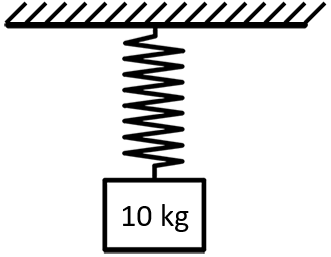## Spring Stretch

Consider a 10 kg object stretches a spring 0.25 m. If the object were swapped out with a larger 15 kg one, how many meters would the spring stretch?Hint
A spring’s deflection and force are related by:
$$F=kx$$$where $$k$$ is the spring constant and $$x$$ is the deflection. Hint 2 First solve for the spring constant with the lower weight. Then, use the spring constant to determine the deflection via the same equation. A spring’s deflection and force are related by: $$F=kx$$$
where $$k$$ is the spring constant and $$x$$ is the deflection.
Since $$Force=mass \times a$$ , we need to multiply the mass by acceleration due to gravity for both objects:
$$F_1=10kg\times 9.8m/s^2=98\:N$$$$$F_2=15kg\times 9.8m/s^2=147\:N$$$
The spring constant is the same for both scenarios, so let’s calculate $$k$$ for the lower weight because there is only one unknown variable:
$$k=\frac{F_1}{x_1}=\frac{98N}{0.25m}=392\:N/m$$$Next, let’s solve for the spring stretch with the larger weight using the spring constant we just determined: $$x_2=\frac{F_2}{k}=\frac{147N}{392N/m}=0.375\:m$$$
Alternatively, we could have solved this problem more quickly using a proportional relationship since the spring constant and gravity are the same in both scenarios:
$$\frac{m_1}{x_1}=\frac{k}{g}=\frac{m_2}{x_2}$$$$$\frac{10kg}{0.25m}=\frac{15kg}{x_2}$$$
$$x_2=\frac{15kg(0.25m)}{10kg}=\frac{3.75m}{10}=0.375\:m$$\$
0.375 m